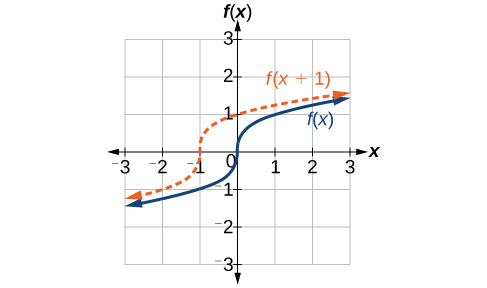# 1.5 Transformation of functions  (Page 2/22)

 Page 2 / 22

Given a tabular function, create a new row to represent a vertical shift.

1. Identify the output row or column.
2. Determine the magnitude of the shift.
3. Add the shift to the value in each output cell. Add a positive value for up or a negative value for down.

## Shifting a tabular function vertically

A function $\text{\hspace{0.17em}}f\left(x\right)\text{\hspace{0.17em}}$ is given in [link] . Create a table for the function $\text{\hspace{0.17em}}g\left(x\right)=f\left(x\right)-3.$

 $x$ 2 4 6 8 $f\left(x\right)$ 1 3 7 11

The formula $\text{\hspace{0.17em}}g\left(x\right)=f\left(x\right)-3\text{\hspace{0.17em}}$ tells us that we can find the output values of $\text{\hspace{0.17em}}g\text{\hspace{0.17em}}$ by subtracting 3 from the output values of $\text{\hspace{0.17em}}f.\text{\hspace{0.17em}}$ For example:

Subtracting 3 from each $\text{\hspace{0.17em}}f\left(x\right)\text{\hspace{0.17em}}$ value, we can complete a table of values for $\text{\hspace{0.17em}}g\left(x\right)\text{\hspace{0.17em}}$ as shown in [link] .

 $x$ 2 4 6 8 $f\left(x\right)$ 1 3 7 11 $g\left(x\right)$ −2 0 4 8

The function $\text{\hspace{0.17em}}h\left(t\right)=-4.9{t}^{2}+30t\text{\hspace{0.17em}}$ gives the height $\text{\hspace{0.17em}}h\text{\hspace{0.17em}}$ of a ball (in meters) thrown upward from the ground after $\text{\hspace{0.17em}}t\text{\hspace{0.17em}}$ seconds. Suppose the ball was instead thrown from the top of a 10-m building. Relate this new height function $\text{\hspace{0.17em}}b\left(t\right)\text{\hspace{0.17em}}$ to $\text{\hspace{0.17em}}h\left(t\right),\text{\hspace{0.17em}}$ and then find a formula for $\text{\hspace{0.17em}}b\left(t\right).$

$b\left(t\right)=h\left(t\right)+10=-4.9{t}^{2}+30t+10$

## Identifying horizontal shifts

We just saw that the vertical shift is a change to the output, or outside, of the function. We will now look at how changes to input, on the inside of the function, change its graph and meaning. A shift to the input results in a movement of the graph of the function left or right in what is known as a horizontal shift , shown in [link] .Horizontal shift of the function   f ( x ) = x 3 .   Note that   h = + 1   shifts the graph to the left, that is, towards negative values of   x .

For example, if $\text{\hspace{0.17em}}f\left(x\right)={x}^{2},\text{\hspace{0.17em}}$ then $\text{\hspace{0.17em}}g\left(x\right)={\left(x-2\right)}^{2}\text{\hspace{0.17em}}$ is a new function. Each input is reduced by 2 prior to squaring the function. The result is that the graph is shifted 2 units to the right, because we would need to increase the prior input by 2 units to yield the same output value as given in $\text{\hspace{0.17em}}f.$

## Horizontal shift

Given a function $\text{\hspace{0.17em}}f,\text{\hspace{0.17em}}$ a new function $\text{\hspace{0.17em}}g\left(x\right)=f\left(x-h\right),\text{\hspace{0.17em}}$ where $\text{\hspace{0.17em}}h\text{\hspace{0.17em}}$ is a constant, is a horizontal shift    of the function $\text{\hspace{0.17em}}f.\text{\hspace{0.17em}}$ If $\text{\hspace{0.17em}}h\text{\hspace{0.17em}}$ is positive, the graph will shift right. If $\text{\hspace{0.17em}}h\text{\hspace{0.17em}}$ is negative, the graph will shift left.

## Adding a constant to an input

Returning to our building airflow example from [link] , suppose that in autumn the facilities manager decides that the original venting plan starts too late, and wants to begin the entire venting program 2 hours earlier. Sketch a graph of the new function.

We can set $\text{\hspace{0.17em}}V\left(t\right)\text{\hspace{0.17em}}$ to be the original program and $\text{\hspace{0.17em}}F\left(t\right)\text{\hspace{0.17em}}$ to be the revised program.

In the new graph, at each time, the airflow is the same as the original function $\text{\hspace{0.17em}}V\text{\hspace{0.17em}}$ was 2 hours later. For example, in the original function $\text{\hspace{0.17em}}V,\text{\hspace{0.17em}}$ the airflow starts to change at 8 a.m., whereas for the function $\text{\hspace{0.17em}}F,\text{\hspace{0.17em}}$ the airflow starts to change at 6 a.m. The comparable function values are $\text{\hspace{0.17em}}V\left(8\right)=F\left(6\right).\text{\hspace{0.17em}}$ See [link] . Notice also that the vents first opened to at 10 a.m. under the original plan, while under the new plan the vents reach at 8 a.m., so $\text{\hspace{0.17em}}V\left(10\right)=F\left(8\right).$

In both cases, we see that, because $\text{\hspace{0.17em}}F\left(t\right)\text{\hspace{0.17em}}$ starts 2 hours sooner, $\text{\hspace{0.17em}}h=-2.\text{\hspace{0.17em}}$ That means that the same output values are reached when $\text{\hspace{0.17em}}F\left(t\right)=V\left(t-\left(-2\right)\right)=V\left(t+2\right).$

what is set?
a colony of bacteria is growing exponentially doubling in size every 100 minutes. how much minutes will it take for the colony of bacteria to triple in size
I got 300 minutes. is it right?
Patience
no. should be about 150 minutes.
Jason
It should be 158.5 minutes.
Mr
ok, thanks
Patience
100•3=300 300=50•2^x 6=2^x x=log_2(6) =2.5849625 so, 300=50•2^2.5849625 and, so, the # of bacteria will double every (100•2.5849625) = 258.49625 minutes
Thomas
what is the importance knowing the graph of circular functions?
can get some help basic precalculus
What do you need help with?
Andrew
how to convert general to standard form with not perfect trinomial
can get some help inverse function
ismail
Rectangle coordinate
how to find for x
it depends on the equation
Robert
yeah, it does. why do we attempt to gain all of them one side or the other?
Melissa
whats a domain
The domain of a function is the set of all input on which the function is defined. For example all real numbers are the Domain of any Polynomial function.
Spiro
Spiro; thanks for putting it out there like that, 😁
Melissa
foci (–7,–17) and (–7,17), the absolute value of the differenceof the distances of any point from the foci is 24.
difference between calculus and pre calculus?
give me an example of a problem so that I can practice answering
x³+y³+z³=42
Robert
dont forget the cube in each variable ;)
Robert
of she solves that, well ... then she has a lot of computational force under her command ....
Walter
what is a function?
I want to learn about the law of exponent
explain this
what is functions?
A mathematical relation such that every input has only one out.
Spiro
yes..it is a relationo of orders pairs of sets one or more input that leads to a exactly one output.
Mubita
Is a rule that assigns to each element X in a set A exactly one element, called F(x), in a set B.
RichieRich

#### Get Jobilize Job Search Mobile App in your pocket Now!ByByBy OpenStaxBy David GeltnerBy OpenStaxBy Angela JanuaryBy RhodesBy OpenStaxBy Anh DaoBy Brooke DelaneyBy Brooke DelaneyBy Yasser Ibrahim